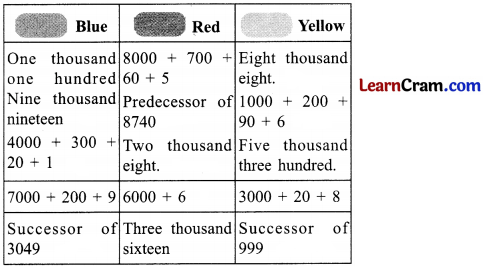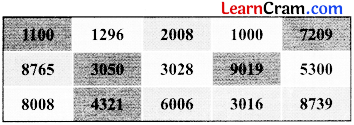# DAV Class 3 Maths Chapter 1 Brain Teasers Solutions

The DAV Class 3 Maths Book Solutions and DAV Class 3 Maths Chapter 1 Brain Teasers Solutions of Numbers upto 9999 offer comprehensive answers to textbook questions.

## DAV Class 3 Maths Ch 1 Brain Teasers Solutions

Question 1.

(a) The numeral for nine thousand ninety is _______.
(i) 9900
(ii) 9090
(iii) 9009
(iv) 0909
Solution:
(ii) 9090
The numeral for nine thousand ninety is 9090.
So Ans. is (ii)

(b) There are _______ tens in one hundred.
(i) 0
(ii) 1
(iii) 100
(iv) 10
Solution:
There are 10 tens in one hundred.
So Ans. is (iv)(c) The numeral for 5000 + 20 + 4 is _________
(i) 5204
(ii) 5024
(iii) 524
(iv) 542
Solution:
(ii) 5024
The numeral for 5000 + 20 + 4 is 5024
So Ans. is (ii).

(d) If the places of the digits 8 and 6 are interchanged in the numeral 8176, then the new numeral is
(i) 1786
(ii) 6178
(iii) 7168
(iv) 7861
Solution:
(ii) 6178
Places of 8 and 6 are interchanged
New numeral = 6178
So Ans. is (ii)

(e) Which place should we compare to determine the greater of the given numerals – 7295 and 7259?
(i) ones
(ii) hundreds
(iii) tens
(iv) thousands
Solution:
(iii) tens
We compare tens place.
So Ans. is (iii)Question 2.
Find these numbers in the given chart. Colour them using pencil colours or crayons.Chart:Solution:Question 3.
Encircle the numbers in which place value of 8 is 80.
358, 2681, 183, 86, 843, 8326, 486, 8
Solution:
358, 2681, 183, 86, 843, 8326, 486, 8

Question 4.
Write the smallest 4-digit number using the digits, 4, 1, 8 and 0 only once.
Solution:
1048

Question 5.
Write the greatest 3-digit number using the digits 9, 0 and 3 only once.
Solution:
930.

Question 6.
Complete the pattern.

(a) 1005, 1015, 1025, ____, ____, ____.
Solution:
1005, 1015, 1025, 1035, 1045, 1055

(b) 2222, 3333, 4444, ____, ____, ____.
Solution:
2222, 3333, 4444, 5555, 6666, 7777

Question 7.
Fill in the blanks.

(a) The expanded form of 3783 is ______.
Solution:
The expanded form of 3783 is 13000 + 700 + 80 + 3

(b) The greatest 4-digit number is ______.
Solution:
The greatest 4-digit number is “9999”.

(c) Place value of 6 in 5628 is ______.
Solution:
Place value of 6 in 5628 is 6 hundreds or 600 digits

(d) The predecessor of smallest 3-digit number has ______ digits.
Solution:
The predecessor of smallest 3 – digit number has 2 digits.Question 1.
Write the numbers that follow:

(a) 1034 ____ ____ ____ ____ ____
Solution:
1034 1035 1036 1037 1038 1039

(b) 3647 ____ ____ ____ ____ ____
Solution:
3647 3648 3649 3650 3651 3652

Question 2.
Write in expanded form.

(a) 4573 = _______
Solution:
4573 = 4000 + 500 + 70 + 3

(b) 9701 = _______
Solution:
9701 = 9000 + 700 + 0 + 1

(c) 6832 = _______
Solution:
6832 = 6000 + 800 + 30 + 2

Question 3.
Make the greatest and smallest 3-digit numbers with given digits.

(a) 8, 3, 6
Solution:
Digits 8, 3, 6
Smallest number 3, 6, 8
Greatest number 8, 6, 3

(b) 4, 0, 2
Solution:
Digits 4, 0, 2
Smallest number 2, 0, 4
Greatest number 4, 2, 0Question 4.
Write >, < or =

(a) 9378 ____ 999
Solution:
9378 > 999

(b) 7307 ____ 7037
Solution:
7307 > 7037

(c) 638 ____ 128
Solution:
638 > 128

(d) 1720 ____ 1270
Solution:
1720 > 1270

(e) 107 ____ 170
Solution:
107 < 170

(f) 111 ____ 111
Solution:
111 = 111Question 5.
Write (a) in descending order and (b) in ascending order.

(a) 4678, 3050, 5860, 7312, 6333
Solution:
Descending order = 7312, 6333, 5860, 4678, 3050

(b) 6030, 2015, 1005, 1075, 2005
Solution:
Ascending order = 1005, 1075, 2005, 2015, 6030